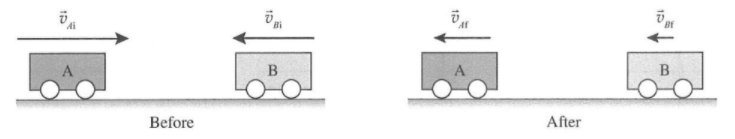# Problem: Two carts roll toward each other on a level table. The vectors represent the velocities of the carts just before and just after they collide. a. Draw and label a vector for each cart to represent the change in velocity from before to after the collision. Make the magnitude and direction of your vectors consistent with the vectors drawn aboveb. How does the direction of the average acceleration of cart A compare to the direction of the average acceleration of cart B over the time interval shown? Explain. c. For the time interval shown, is the magnitude of the average acceleration of cart A greater than, less than, or equal to the magnitude of the average acceleration of cart B? Explain

###### FREE Expert Solution

The change in velocity before and after the collision can be found by the following:

For cart A:

$\overline{){\mathbf{∆}}{{\mathbf{v}}}_{{\mathbf{A}}}{\mathbf{=}}{{\mathbf{v}}}_{\mathbf{A}\mathbf{f}}{\mathbf{-}}{{\mathbf{v}}}_{{\mathbf{Ai}}}}$

For cart B:

$\overline{){\mathbf{∆}}{{\mathbf{v}}}_{{\mathbf{B}}}{\mathbf{=}}{{\mathbf{v}}}_{\mathbf{B}\mathbf{f}}{\mathbf{-}}{{\mathbf{v}}}_{{\mathbf{Bi}}}}$

a.

The vectors of the change in velocity of the two carts are then drawn as below.

100% (202 ratings)###### Problem Details

Two carts roll toward each other on a level table. The vectors represent the velocities of the carts just before and just after they collide.a. Draw and label a vector for each cart to represent the change in velocity from before to after the collision. Make the magnitude and direction of your vectors consistent with the vectors drawn above

b. How does the direction of the average acceleration of cart A compare to the direction of the average acceleration of cart B over the time interval shown? Explain.

c. For the time interval shown, is the magnitude of the average acceleration of cart A greater than, less than, or equal to the magnitude of the average acceleration of cart B? Explain